Orbiter-Forum[SDK Question] Trouble with the produced drag of airfoils
 Register Blogs Orbinauts List Social Groups FAQ Projects Mark Forums Read

 Orbiter SDK Orbiter software developers post your questions and answers about the SDK, the API interface, LUA, meshing, etc.03-29-2019, 09:46 AM #1 DerNerf Orbinaut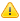Trouble with the produced drag of airfoils Hello everyone, I'm currently (still) working on a lifting capsule Soyuz model for re-entry simulation. As described in the API Guide I have defined two airfoils, one for horizontal and one for vertical lift with all the given parameters. The Code is as follows: Code: ```double D1 = 2.2; VECTOR3 crossSecVec; crossSecVec.x = 4.052293854; // projection along x-axis into yz-plane crossSecVec.y = 4.052293854; // projection along y-axis into xz-plane crossSecVec.z = PI * ((D1 * D1) / 4); SetCrossSections(crossSecVec); ClearAirfoilDefinitions(); hVertAirfoil = CreateAirfoil2( LIFT_VERTICAL, dyn->CoP, SoyuzCMAerodynamicCoeffVert, 2.142, 0, D1 ); hHoriAirfoil = CreateAirfoil2( LIFT_HORIZONTAL, dyn->CoP, SoyuzCMAerodynamicCoeffHoriz, 2.142, 0, D1 );``` The Callback function for the coefficients works fine and has the coefficients interpolated from literature values. With this the capsule is aerdoynamicly stable and flies just as it should. However the Lift-to-Drag ratio is at all points of the simulation half the size it should be (compared to the values given by literature) . Calcluating the drag manually for some points of the trajectory shows, that the drag is twice as high as supposed. Thus the Lift is just fine as it is. Since the difference in Lift and Drag calculation is simply the coefficient I tried working with them to solve the problem. Dividing the drag coefficient in half for each airfoil as well as setting one of them to zero seems to effect the whole aerodynmaic model causing the capsule to fly at a high slip angle of ~45° rather than the supposed one of ~0°. The angle of attack is also way higher than the old value of 17,5° (for the working model with twice the drag) Does anyone have an idea of why this is happening and how to solve the problem? I personally have run out of ideas. Changes in the chord length and wing span have, as expected, no influence on the Lift-to-Drag ratio. Thanks in advance for your time and effort03-29-2019, 10:01 AM #2 Urwumpe Not funny anymore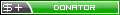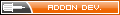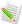What is your lift function?03-29-2019, 11:26 AM #3 DerNerf OrbinautWhat do you mean by lift function? The Coefficients are calculated this way: Code: ```/* Mach Number Steps of Reference Points */ const double aero_MAref = { 0.60, 0.95, 1.10, 1.78, 2.52, 5.96 }; /* Angle of Attack (AoA) Steps of Reference Points */ const double aero_AOAref = { 0.0*RAD, 5.0*RAD, 10.0*RAD, 15.0*RAD, 20.0*RAD, 25.0*RAD, 30.0*RAD }; /* Axial Force Coefficient (This is NOT equal to Drag) for |aoa| = { 0, 5, 10, 15, 20, 25, 30 } deg and for all reference mach numbers. */ const double aero_cx = { /* |aoa| 0 5 10 15 20 25 30 deg*/ { 1.01, 1.00, 1.00, 0.975, 0.958, 0.921, 0.879 }, // Ma = 0.60 { 1.27, 1.27, 1.25, 1.22, 1.21, 1.18, 1.15 }, // Ma = 0.95 { 1.40, 1.40, 1.39, 1.37, 1.34, 1.29, 1.25 }, // Ma = 1.10 { 1.52, 1.52, 1.50, 1.46, 1.43, 1.38, 1.31 }, // Ma = 1.78 { 1.52, 1.52, 1.48, 1.46, 1.43, 1.38, 1.31 }, // Ma = 2.52 { 1.57, 1.54, 1.51, 1.44, 1.38, 1.28, 1.18 }, // Ma = 5.96 }; /* Normal Force Coefficient (This is NOT equal to Lift) for |aoa| = { 0, 5, 10, 15, 20, 25, 30 } deg and for all reference mach numbers. */ const double aero_cz = { /* |aoa| 0 5 10 15 20 25 30 deg*/ { 0.00, 0.04, 0.06, 0.07, 0.06, 0.04, 0.02 }, // Ma = 0.60 { 0.00, 0.03, 0.04, -0.01, -0.01, -0.07, -0.14 }, // Ma = 0.95 { 0.00, 0.03, 0.04, 0.00, -0.04, -0.09, -0.13 }, // Ma = 1.10 { 0.00, 0.03, -0.01, -0.08, -0.16, -0.26, -0.36 }, // Ma = 1.78 { 0.00, -0.04, -0.09, -0.14, -0.18, -0.24, -0.31 }, // Ma = 2.52 { 0.00, -0.03, -0.06, -0.09, -0.13, -0.19, -0.25 }, // Ma = 5.96 }; /* Lift to Drag Ratio for |aoa| = { 0, 5, 10, 15, 20, 25, 30 } deg and for all reference mach numbers. */ const double aero_ld = { /* |aoa| 0 5 10 15 20 25 30 deg*/ { 0.00, 0.123, 0.249, 0.343, 0.443, 0.514, 0.614 }, // Ma = 0.60 { 0.00, 0.100, 0.209, 0.286, 0.363, 0.389, 0.420 }, // Ma = 0.95 { 0.00, 0.100, 0.200, 0.271, 0.323, 0.389, 0.446 }, // Ma = 1.10 { 0.00, 0.066, 0.171, 0.200, 0.229, 0.243, 0.257 }, // Ma = 1.78 { 0.00, 0.057, 0.114, 0.171, 0.229, 0.271, 0.300 }, // Ma = 2.52 { 0.00, 0.063, 0.129, 0.191, 0.257, 0.300, 0.329 }, // Ma = 5.96 }; /* Pitch Moment Coefficient for |aoa| = { 0, 5, 10, 15, 20, 25, 30 } deg and for all reference mach numbers. */ const double aero_cm = { /* |aoa| 0 5 10 15 20 25 30 deg*/ { -0.0300, -0.0200, -0.0100, +0.0035, +0.0153, +0.0247, +0.0318 }, // Ma = 0.60 { -0.0359, -0.0241, -0.0141, -0.0059, +0.0012, +0.00711, +0.0094 }, // Ma = 0.95 { -0.0388, -0.0282, -0.0188, -0.0094, -0.0012, +0.0053, +0.0082 }, // Ma = 1.10 { -0.0447, -0.0400, -0.0300, -0.0176, -0.0041, +0.0082, +0.0112 }, // Ma = 1.78 { -0.0500, -0.0412, -0.0300, -0.0176, -0.0041, +0.0082, +0.0112 }, // Ma = 2.52 { -0.0535, -0.0435, -0.0329, -0.0224, -0.0118, +0.0000, +0.0100 } // Ma = 5.96 }; void SoyuzCMAerodynamicCoeffVert(double aoa, double Ma, double Re, double *cl, double *cm, double *cd) { /* We enter backwards, so rotate the AoA by PI */ double aoa2 = 0; if ( aoa < 0) aoa2 = -(aoa + PI); if ( aoa > 0) aoa2 = -(aoa - PI); double aoa3 = aoa2; //the actual AoA is needed for the correct lift and drag Coeffiecents aoa2 = abs (aoa2 ); // use only absolute value here /* Get lower AoA index */ int i_AoA1=-1, i_AoA2=0; double d_AoA; // 0 .. 1 point between i_AoA1 and i_AoA2 for (int i=0; i<7; i++) if (aero_AOAref[i] < aoa2){ i_AoA1 = i;} else break; i_AoA2 = i_AoA1 + 1; d_AoA = (aoa2 - aero_AOAref[i_AoA1]) / (aero_AOAref[i_AoA2] - aero_AOAref[i_AoA1]); /* If AoA is higher than 30 deg, limit.*/ if (aoa2 > aero_AOAref) { i_AoA1 = 5; i_AoA2 = 6; d_AoA = 1; } if (aoa3 > 30 * (PI / 180)) aoa3 = 30 * (PI / 180); else if (aoa3 < -30 * (PI / 180)) aoa3 = -30 * (PI / 180); /* Get next lower mach number index */ int i_Ma1=-1, i_Ma2=0; double d_Ma = 0; // 0 .. 1 point between i_Ma1 and i_Ma2 for (int i=0; i<6; i++) if (aero_MAref[i] aero_MAref) { i_Ma1 = 4; i_Ma2 = 5; d_Ma = 1; } /* If mach number is lower, set to the lowest mach index instead of extrapolation.*/ if (i_Ma1 == -1) { i_Ma1 = 0; i_Ma2 = 1; d_Ma = 0; } /*Calculation of the actual pure axial/normal Coefficient*/ /* Axial Component */ double cx11 = aero_cx[i_Ma1][i_AoA1]; // lower Ma, lower AoA double cx21 = aero_cx[i_Ma2][i_AoA1]; // higher Ma, lower AoA double cx12 = aero_cx[i_Ma1][i_AoA2]; // lower Ma, higher AoA double cx22 = aero_cx[i_Ma2][i_AoA2]; // higher Ma, higher AoA /* Linear interpolation over Ma */ double cx1 = cx11 + d_Ma * (cx21 - cx11); // for lower AoA double cx2 = cx12 + d_Ma * (cx22 - cx12); // for higher AoA /* Linear interpolation over AoA */ double cx = cx1 + d_AoA * (cx2 - cx1); /* Normal Component */ double cz11 = aero_cz[i_Ma1][i_AoA1]; // lower Ma, lower AoA double cz21 = aero_cz[i_Ma2][i_AoA1]; // higher Ma, lower AoA double cz12 = aero_cz[i_Ma1][i_AoA2]; // lower Ma, higher AoA double cz22 = aero_cz[i_Ma2][i_AoA2]; // higher Ma, higher AoA /* Linear interpolation over Ma */ double cz1 = cz11 + d_Ma * (cz21 - cz11); // for lower AoA double cz2 = cz12 + d_Ma * (cz22 - cz12); // for higher AoA /* Linear interpolation over AoA */ double cz = cz1 + d_AoA * (cz2 - cz1); /*Drag and Lift Coefficient*/ *cd = cx * cos(aoa3) + cz * sin(aoa3); //*cd = *cd/2; *cl = -cx * sin(aoa3) + cz * cos(aoa3); *cl *= -1; // we fly backward in our coordinate system, so reverse sign /* Pitch Moment Coefficient */ double cm11 = aero_cm[i_Ma1][i_AoA1]; // lower Ma, lower AoA double cm21 = aero_cm[i_Ma2][i_AoA1]; // higher Ma, lower AoA double cm12 = aero_cm[i_Ma1][i_AoA2]; // lower Ma, higher AoA double cm22 = aero_cm[i_Ma2][i_AoA2]; // higher Ma, higher AoA /* Linear interpolation over Ma */ double cm1 = cm11 + d_Ma * (cm21 - cm11); // for lower AoA double cm2 = cm12 + d_Ma * (cm22 - cm12); // for higher AoA /* Linear interpolation over AoA */ *cm = cm1 + d_AoA * (cm2 - cm1); *cm *= -1; // we fly backward in our coordinate system, so reverse sign if (aoa < 0) *cm *= -1; // for negative angles of attack, use different sign } void SoyuzCMAerodynamicCoeffHoriz(double aoa, double Ma, double Re, double *cl, double *cm, double *cd) { /* We enter backwards, so rotate the AoA by PI */ double aoa2 = 0; if (aoa < 0) aoa2 = -(aoa + PI); if (aoa > 0) aoa2 = -(aoa - PI); double aoa3 = aoa2; //the actual AoA is needed for the correct lift and drag Coeffiecents aoa2 = abs(aoa2); // use only absolute value here /* Get lower AoA index */ int i_AoA1 = -1, i_AoA2 = 0; double d_AoA; // 0 .. 1 point between i_AoA1 and i_AoA2 for (int i = 0; i<7; i++) if (aero_AOAref[i] < aoa2){ i_AoA1 = i; } else break; i_AoA2 = i_AoA1 + 1; d_AoA = (aoa2 - aero_AOAref[i_AoA1]) / (aero_AOAref[i_AoA2] - aero_AOAref[i_AoA1]); /* If AoA is higher than 30 deg, limit.*/ if (aoa2 > aero_AOAref) { i_AoA1 = 5; i_AoA2 = 6; d_AoA = 1; } if (aoa3 > 30 * (PI / 180)) aoa3 = 30 * (PI / 180); else if (aoa3 < -30 * (PI / 180)) aoa3 = -30 * (PI / 180); /* Get next lower mach number index i_Ma==-1 oder i_Ma2>5 bedeutet extrapolation */ int i_Ma1 = -1, i_Ma2 = 0; double d_Ma = 0; // 0 .. 1 point between i_Ma1 and i_Ma2 for (int i = 0; i<6; i++) if (aero_MAref[i] aero_MAref) { i_Ma1 = 4; i_Ma2 = 5; d_Ma = 1; } /* If mach number is lower, set to the lowest mach index instead of extrapolation. */ if (i_Ma1 == -1) { i_Ma1 = 0; i_Ma2 = 1; d_Ma = 0; } /* Axial Component (Drag) */ double cx11 = aero_cx[i_Ma1][i_AoA1]; // lower Ma, lower AoA double cx21 = aero_cx[i_Ma2][i_AoA1]; // higher Ma, lower AoA double cx12 = aero_cx[i_Ma1][i_AoA2]; // lower Ma, higher AoA double cx22 = aero_cx[i_Ma2][i_AoA2]; // higher Ma, higher AoA /* Linear interpolation over Ma */ double cx1 = cx11 + d_Ma * (cx21 - cx11); // for lower AoA double cx2 = cx12 + d_Ma * (cx22 - cx12); // for higher AoA /* Linear interpolation over AoA */ double cx = cx1 + d_AoA * (cx2 - cx1); /* Normal Component (Lift) */ double cz11 = aero_cz[i_Ma1][i_AoA1]; // lower Ma, lower AoA double cz21 = aero_cz[i_Ma2][i_AoA1]; // higher Ma, lower AoA double cz12 = aero_cz[i_Ma1][i_AoA2]; // lower Ma, higher AoA double cz22 = aero_cz[i_Ma2][i_AoA2]; // higher Ma, higher AoA /* Linear interpolation over Ma */ double cz1 = cz11 + d_Ma * (cz21 - cz11); // for lower AoA double cz2 = cz12 + d_Ma * (cz22 - cz12); // for higher AoA /* Linear interpolation over AoA */ double cz = cz1 + d_AoA * (cz2 - cz1); /*Drag and Lift Coefficient calculation*/ *cd = cx * cos(aoa3) + cz * sin(aoa3); //*cd = *cd/2; *cl = -cx * sin(aoa3) + cz * cos(aoa3); *cl *= -1; // we fly backward in our coordinate system, so reverse sign /* Pitch Moment Coefficient */ double cm11 = aero_cm[i_Ma1][i_AoA1]; // lower Ma, lower AoA double cm21 = aero_cm[i_Ma2][i_AoA1]; // higher Ma, lower AoA double cm12 = aero_cm[i_Ma1][i_AoA2]; // lower Ma, higher AoA double cm22 = aero_cm[i_Ma2][i_AoA2]; // higher Ma, higher AoA /* Linear interpolation over Ma */ double cm1 = cm11 + d_Ma * (cm21 - cm11); // for lower AoA double cm2 = cm12 + d_Ma * (cm22 - cm12); // for higher AoA /* Linear interpolation over AoA */ *cm = cm1 + d_AoA * (cm2 - cm1); *cm *= -1; // we fly backward in our coordinate system, so reverse sign if (aoa < 0) *cm *= -1; // for negative angles of attack, use different sign }```03-29-2019, 12:03 PM #4 martins Orbiter Founder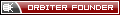This is a stab in the dark, but is it possible that the problem is that both your airfoils are generating drag, but (effectively) only one is generating nonzero lift?03-29-2019, 12:41 PM #5 DerNerf OrbinautQuote: Originally Posted by martins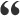This is a stab in the dark, but is it possible that the problem is that both your airfoils are generating drag, but (effectively) only one is generating nonzero lift? Well the lift changes direction with a change of the bank angle, so i would suppose both airfoils do generate lift, as that is, from my understanding, the whole reason to have two airfoils for horizontal and vertical lift. Or do you mean, that the two airfoils create the amount of lift that only one should (compared to the drag they produce)? Is there a way to get the value of the Wing Area that's calculated from the cross sections that the airfoil works with? Like a GetWingArea() type of function that returns the wing area for that moment with the current attitute of the sapcecraft? Then I could manually check, if at least the amount of lift is correct. Changing the Lift would only be a temporary solution for me, since the drag value is obviously not what it should be and, as this is for my Bachlor Thesis, it's fairly important that I work with the real figures. Thanks for the Idea though03-29-2019, 12:50 PM #6 Urwumpe Not funny anymoreQuote: Originally Posted by DerNerfWell the lift changes direction with a change of the bank angle, so i would suppose both airfoils do generate lift, as that is, from my understanding, the whole reason to have two airfoils for horizontal and vertical lift. Actually not. Its a coordinate system that does not care about the bank angle. vertical and horizontal refer to body coordinates, but horizon/surface coordinates. That is why you only find AOA or sideslip angle as polar coordinates, not the bank angle there.03-29-2019, 01:51 PM #7 DerNerf OrbinautQuote: Originally Posted by UrwumpeActually not. Its a coordinate system that does not care about the bank angle. vertical and horizontal refer to body coordinates, but horizon/surface coordinates. That is why you only find AOA or sideslip angle as polar coordinates, not the bank angle there. Okay, I got that. The Coordinate Systems "turn" with the vehicle as it turns and changes its bank anlge. Is it right then, that the vertical lift is from the AoA and the horizontal from the slip angle? The (real) Soyuz is not only aerodynamicly stable but also stabilized by a control system. The Slip angle during re-entry is very small and doen't really exceed +- 2°. That induces very little Lift. If I was willing to simply "ignore" that small horitzontal lift, couldn't I remove the Airfoil with Horizontal lift? Then the drag coming from just one arifoil should have the value I need and the Lift almost stays the same, as most of it is vertical anyways.03-29-2019, 02:14 PM #8 martins Orbiter FounderThe point I was making is that if you have a body that is rotationally symmetric w.r.t. its longitudinal axis (as I guess your capsule approximately is) then your two airfoils probably will also have identical and symmetric lift characteristics. Therefore the body's rotation along its longitudinal axis shouldn't change the lift (except that is rotates in the local vessel frame). So without loss of generality, in the case of negligible slip angle during entry, you can assume that the capsule is rotated such that one of the airfoils is generating all the lift, while the other is generating zero lift. Rotate the capsule by 90 degrees, and it's the other airfoil that is now generating the lift. Anywhere in between, both airfoils generate part of the lift, but the magnitude of the resulting lift vector remains the same. This is what I meant when saying that effectively only one is generating lift. Whereas the drag force from both airfoils is added up in all orientations. So I would argue that the desired drag characteristics must be split evenly over the two airfoils (except induced drag, since that is directly linked to the lift), while each of the airfoils must be defined with the full lift characteristics. Does this make sense? Not sure if my argument is very clear.03-29-2019, 02:38 PM #9 Urwumpe Not funny anymoreI am not sure if it would work with just one foil - the horizontal lift function also has a stabilizing function. You could describe it as zero-lift for simplification, but I would not ignore the torque function.03-29-2019, 05:06 PM #10 DerNerf OrbinautQuote: Originally Posted by martinsThe point I was making is that if you have a body that is rotationally symmetric w.r.t. its longitudinal axis (as I guess your capsule approximately is) then your two airfoils probably will also have identical and symmetric lift characteristics. Therefore the body's rotation along its longitudinal axis shouldn't change the lift (except that is rotates in the local vessel frame). So without loss of generality, in the case of negligible slip angle during entry, you can assume that the capsule is rotated such that one of the airfoils is generating all the lift, while the other is generating zero lift. Rotate the capsule by 90 degrees, and it's the other airfoil that is now generating the lift. Anywhere in between, both airfoils generate part of the lift, but the magnitude of the resulting lift vector remains the same. This is what I meant when saying that effectively only one is generating lift. Whereas the drag force from both airfoils is added up in all orientations. So I would argue that the desired drag characteristics must be split evenly over the two airfoils (except induced drag, since that is directly linked to the lift), while each of the airfoils must be defined with the full lift characteristics. Does this make sense? Not sure if my argument is very clear. Yeah, that makes sense. That could be the case. That would mean we found a part of the problems cause, but still not the solution. Quote: Originally Posted by UrwumpeI am not sure if it would work with just one foil - the horizontal lift function also has a stabilizing function. You could describe it as zero-lift for simplification, but I would not ignore the torque function. Okay, that gave me an Idea. I think I'll try to give the horizontal function a lift and drag coefficient of zero. maybe the fact that there was no "horizontal drag" ment, that the small horizontal lift offset the whole capsule resulting in the weird attitude it was flying at in the end. Thank you very much for your help, I'll let you know if the changes helped ---------- Post added at 05:06 PM ---------- Previous post was at 03:28 PM ---------- Quote: Originally Posted by DerNerfOkay, that gave me an Idea. I think I'll try to give the horizontal function a lift and drag coefficient of zero. maybe the fact that there was no "horizontal drag" ment, that the small horizontal lift offset the whole capsule resulting in the weird attitude it was flying at in the end. Thank you very much for your help, I'll let you know if the changes helped And it did work. She rides smooth as silk just as supposed and the Lift-to-drag ratio is just as it should be for the angle. Once again thank you very much, this helped me greatly And now, onwords to the next thing to fix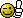Thanked by:03-29-2019, 05:23 PM #11 martins Orbiter FounderGood to hear that it works now! Although the fact that lift and drag for the horizontal foil are now zero makes me slightly ... uneasy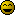What happens if you remove that airfoil altogether? Does it behave the same? Just to confirm: Your lift, moment and drag coefficients _are_ symmetric around AOA=0, correct? That is, cl(aoa=0) = 0 cl(-aoa) = -cl(aoa) same for moment coefficients, and cd(-aoa) = cd(aoa) In that case, if your slip angle is 0, lift will be 0 as well, so it should behave the same as if you force cl=0 outright. What happens if you flip the capsule on its side for the reentry, so that the roles of the horizontal and vertical airfoils are swapped? Does all hell break loose?It might be worth putting a debug output into your airfoil coefficient callback function that echos the input AOA and resulting output cl values on the screen and to monitor that during entry to make sure that the values make sense.Tags airfoil, drag, sdkThread ToolsShow Printable VersionEmail this PagePosting Rules BB code is On Smilies are On [IMG] code is On HTML code is Off You may not post new threads You may not post replies You may not post attachments You may not edit your posts
 Forum Jump User Control Panel Private Messages Subscriptions Who's Online Search Forums Forums Home Orbiter-Forum.com     Announcements     Meets & Greets Orbiter Space Flight Simulator     Orbiter Web Forum         OFMM         Orbiter Forum Space Station         Simpit Forum     General Questions & Help     MFD Questions & Help     Hardware & Software Help     Tutorials & Challenges     Orbiter SDK     Orbiter Visualization Project     Orbiter Beta » Orbiter Project Orbiter Addons     OrbitHangar Addons & Comments     Addons     Addon Development     Addon Requests     Addon Support & Bugs         Addon Developer Forums             Project Apollo - NASSP     Orbiter Lua Scripting Far Side of the Moon     Spaceflight News     Math & Physics     Astronomy & the Night Sky     Backyard Rocketry     Brighton Lounge     International Forum

All times are GMT. The time now is 09:58 PM.